## Introduction

The dielectric insulator is a key component in microelectronic devices such as the central processing unit (CPU), dynamic random-access memory (DRAM) and flash memory. The basic function of the dielectric material is to enhance the capacitive coupling between adjacent metals and semiconductors, although it should also suppress the leakage current between electrodes, which undermines the energy consumption (in CPU and DRAM) or long-term reliability (in flash memory). In past decades, silicon dioxide (SiO2) has been used as an archetypical dielectric material because it allows for defect-free, high-quality thin-film growth. As the integration level of microelectronic devices is currently exponentially increasing, the thickness of SiO2 has decreased to maintain the device performance. However, if the SiO2 layer becomes thinner than ~1 nm, the leakage current due to the quantum tunneling effect begins to dominate,1 which causes serious problems in power consumption and device performance. This technical obstacle has been overcome by replacing SiO2 with insulators that possess high dielectric constants (high-κ).1, 2 With high-κ dielectrics, the dielectric thickness can be increased at the same capacitance, thereby suppressing the leakage current.3 Currently, the favored high-κ dielectrics are HfO2 (as the gate dielectric in CPU),2 ZrO2 (as the capacitor dielectric in DRAM)4 and Al2O3 (as the blocking oxide in charge-trap flash memory).3

In addition to a large dielectric constant, the high-κ dielectric is required to have a large band gap (Eg) to suppress the charge injection from electrodes into dielectrics that cause the leakage current. Therefore, the ideal high-κ dielectrics should possess both large Eg and κ. Notably, when Eg and κ of well-known oxides are plotted (see Figure 1), the trade-off relation is clearly noticeable. That is, materials are abundant with large Eg (>~8 eV) or high κ (>~20); however, no material has been discovered that satisfies both conditions simultaneously. Because this observation is based on a limited set of materials, one may question whether a material possessing both large Eg and κ may indeed exist if the search space is expanded.

Obtaining material information on Eg and κ, particularly the static dielectric constant, would be prohibitive if only experimental measurements were utilized. However, with the recent advances in computational methods and facilities, it is now feasible to compute ab initio various physical properties of the bulk phase in a relatively short period. Recently, several attempts have been made to find an optimal functional material using high-throughput computational screening.5, 6, 7, 8 The machine-learning approach presented by G. Pilania9 is a more accelerated approach to predict properties of large numbers of polymers; however, the prediction accuracy for Eg and κ is low for extending the method to other systems. In this study, we perform ab initio calculations on ~1800 oxides (except for 3d transition metal oxides) that cover most binary and ternary oxides identified to date and suggest novel candidate high-κ dielectrics suitable for each device type. To this end, we set up computational machinery that automatically fetches structures from the database, prepares input files and reliably performs the ab initio calculations.

## Materials and methods

Figure 2 presents a brief scheme of our automation strategy for calculating Eg and κ. First, all of the structures that contain specific cations are collected from the Inorganic Crystal Structure Database.10 We exclude 3d transition metal atoms because the electronic correlation effects are strong and Eg is moderate (<3 eV). The structures are further screened to avoid duplicative calculations on the same structure. The structures with partial atomic occupation are excluded because of the difficulty in computational modeling. In addition to the structures that are stable at ambient conditions, we consider structures that are characterized under high-temperature or high-pressure conditions, provided that they are theoretically stable at zero temperature and pressure conditions, because the metastable structures can exist at ambient conditions through doping11 or in nanocrystalline states.12 The atomic positions and lattice parameters are then relaxed, and the theoretical equilibrium structures are obtained. For the computational code, the Vienna Ab Initio Simulation Package13 is adopted as the core engine for the ab initio calculations. Regarding the exchange-correlation functional between electrons, we employ the generalized gradient approximation (GGA)14 for Eg and local density approximation (LDA)15 for κ. Because GGA and LDA underestimate the band gap, we also perform a hybrid-functional calculation for the band-edge points identified by GGA. The detailed procedure for each step is provided in the Methods section. The reliability of the present automation procedure is confirmed by comparing the extant experimental data with previous calculations.

### Structural relaxation

All experimental structures should first be relaxed theoretically. This step is mandatory because when computing a dielectric constant, the structures are assumed to be at the local equilibrium. The k-points in the first Brillouin zone are carefully and automatically sampled such that the total energy and stress tensor components are converged within 5 meV per atom and 10 kbar, respectively. The energy cutoff for the plane-wave basis set is selected based on the atomic species and pseudopotential types. The structural relaxation is performed until the atomic force and stress tensor are reduced to below 0.02 eV Å−1 and 5 kbar, respectively. Many structures reported at high-temperature or high-pressure conditions often have higher symmetry compared with low-temperature phases. When these structures are relaxed with the symmetry maintained, the final structures often possess unstable phonon modes. We exclude these structures from consideration as new high-κ candidates because of their stability issue.

### Computation of band gap

We first computed energy levels along the lines connecting the high-symmetry k-points within GGA.16 On the basis of the results, the k-points corresponding to the valence top and conduction minimum are determined. Because the band gap underestimation is severe in the semilocal functional, we additionally perform a hybrid-functional (HSE06) calculation17 (without further structural relaxation) and calculate the energy levels at the band-edge k-points identified by GGA (this scheme is called HSE@GGA hereafter). This process assumes that the band structure rigidly shifts upon application of the hybrid functional. Supplementary Figure S1 in the Supplementary Information shows that the band structure from the hybrid functional is approximately a rigid shift of the GGA result. By adopting the HSE@GGA scheme, we could significantly reduce the computational cost compared with the full hybrid-functional calculations. For oxides that include heavy elements such as Tl, Pb and Bi, the spin-orbit coupling is included in computing Eg.

To ensure the reliability of the present scheme, test calculations on several oxides are performed and compared with the experiment and previous GGA calculations (see Figure 3a). The estimated energy gaps are in good agreement with the experiment, although sizeable errors of up to 1 eV are noticeable for oxides with Eg larger than ~4 eV. These errors are due to the fixed fraction of the exact exchange term in the HSE06 functional, which should be increased in the large-gap materials.18 This finding implies that more sophisticated methods such as GW calculations can be utilized to obtain more precise values of Eg. We note that the GW calculations are too expensive to be incorporated into the high-throughput screening. In addition, it is not yet known which level of GW approximations can be universally applied to every class of oxides.19 Nevertheless, the accuracy of HSE@GGA is sufficient to screen promising dielectrics in the present study.

### Computation of dielectric constant

To compute the electronic permittivity, the linear-response method based on the density functional perturbation theory is used to obtain Born effective charges and phonon modes at the zone center.20 Because the linear-response computation is sensitive to the k-point sampling, we double the k-point density along each direction. The static dielectric constant (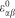) is then calculated using the following formula: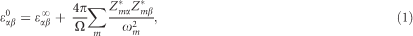where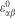, Ω, ωm and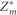denote the dielectric tensor contributed by electrons, the unit-cell volume, the frequency of the infrared-active phonon with the mode number of m and the mode-effective Born effective charges, respectively. The subscripts α and β in equation (1) indicate the directions. The dielectric constant (κ) is obtained by averaging the diagonal components of. The theoretical κ of some oxides determined by GGA and LDA are compared with the experiment (see Figure 3b). The mean absolute errors for materials with κ<30 are 2.06 and 1.37 for the GGA and LDA results, respectively. The GGA results exhibit larger errors than those of LDA because the ionic part of κ is sensitive to the low-frequency phonon modes that are significantly softened when the lattice parameters are expanded in GGA. Therefore, we employ LDA for computing κ.

### Computational cost

The average computational cost was ~70 CPU hours per structure on the 24-core cluster. The most time-consuming part was the hybrid-functional calculation in the HSE@GGA scheme (50%) followed by the density functional perturbation theory calculations for the dielectric constant (27%). The structure relaxation and band-edge searching consumed ~15% and 8% of the total computational time, respectively.

## Results

### Total property map

We calculated 1762 oxides in total and obtained a large property database. Figure 4 presents the property map of Eg versus κ for 1158 binary and ternary oxides. We excluded the structures that were metallic or unstable under the ambient condition according to the results. First, the inverse relation between Eg and κ was roughly valid, and the oxides satisfying the ideal condition were scarce. To select candidate high-κ oxides from the database, we defined a figure of merit for reducing the leakage current. The leakage current density by direct tunneling (JDT) can be expressed using the following semi-empirical formula:21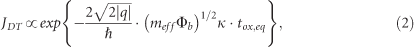where q and meff are the charge and tunneling effective mass, respectively, of the electron or hole, Фb is the injection barrier, and tox,eq represents the equivalent oxide thickness. We define (meff Фb )1/2κ in the exponent as the figure of merit (fFOM), which indicated that the tunneling current is exponentially suppressed with fFOM. One can compute meff and Фb theoretically,22 but the process requires demanding calculations that are not compatible with the high-throughput approach. Here we make a crude but reasonable assumption that the two parameters are roughly proportional to Eg,23 which approximates fFOM as simply Eg·κ. Each point in Figure 4 is color coded according to fFOM.

### New candidate high-κ materials

Among the oxides in Figure 4, c-BeO, the high-pressure phase of BeO in the rocksalt structure (see Figure 5a), is particularly prominent as it has the unusual combination of 10.1 eV and 275 for Eg and κ, respectively. Consequently, its fFOM is considerably beyond those of other materials. The wurtzite BeO (w-BeO), which is stable at ambient conditions, has already been used in dielectric applications as it has the largest band gap among all the oxides. The atomic-layer-deposited w-BeO was fabricated on Si substrates and employed as a diffusion barrier of oxygen between Si and high-κ dielectrics such as HfO2.24 However, w-BeO has a very small κ of ~7. However, c-BeO has not been applied in microelectronic devices to date as far as we are aware. The origin of the large κ of c-BeO is its soft optical phonon mode (~3.5 THz), wherein the Be and O atoms vibrate in opposite directions (see Figures 5a and b). In c-BeO, the Be–O bond length is longer than that in w-BeO by 0.11 Å, which softens the optical phonon mode. The computed total energy indicates that c-BeO is less stable than w-BeO by 0.483 eV per atom. The large energy difference implies that c-BeO would be difficult to stabilize under ambient conditions. However, we pay attention to the experiments in Adelmann et al.25, Kita et al.26 and Tsipas et al.27, which indicate that the high-temperature phases of HfO2 and ZrO2 can be synthesized as thin films by external doping or strain. More importantly, the doped phases possessed increased dielectric constants, as predicted by theory.28 Therefore, we reasonably expect that c-BeO can be stabilized by doping or strain and will exhibit physical properties similar to the present calculations.